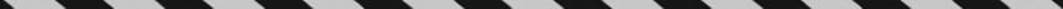﻿ ﻿INTEGER PROBLEMS - Word Problems - ALGEBRA - SAT SUBJECT TEST MATH LEVEL 1

## ALGEBRA## CHAPTER 7Word Problems### INTEGER PROBLEMS

Since the difference between consecutive integers is 1, if n represents an integer, the next three consecutive integers are n + 1, n + 2, and n + 3.

Since the difference between consecutive even integers (or consecutive odd integers) is 2, if n represents an even integer, the next three consecutive even integers are n + 2, n + 4, and n + 6.

EXAMPLE 5: Ruth wrote down three consecutive odd integers. She then multiplied the first one by 2, the second one by 3, and the third one by 4. If the sum of all six numbers is 400, what is the first number she wrote?

Since the first three numbers were consecutive odd integers, represent them by n, n + 2, and n + 4. The other three numbers were 2n, 3(n + 2), and 4(n + 4), so﻿